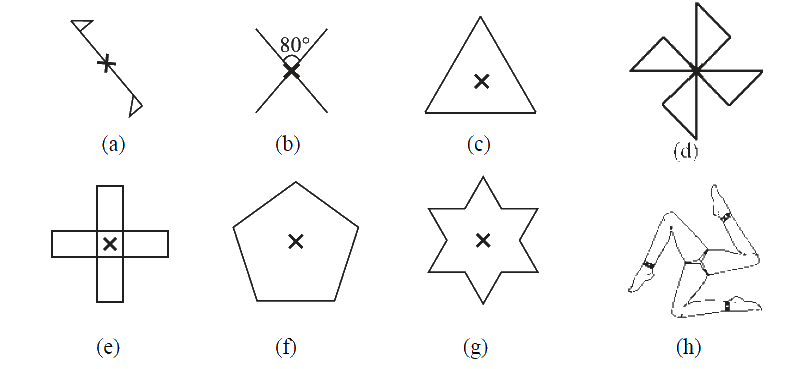# Ex.14.2 Q2 Symmetry - NCERT Maths Class 7

## Question

Give the order of rotational symmetry for each figure:Video Solution
Symmetry
Ex 14.2 | Question 2

## Text Solution

What is known?

Figures.

What is unknown?

The order of rotational symmetry

Reasoning:

To solve this question, remember the concept of rotational symmetry. Rotational symmetry is when an object is rotated around a centre point (turned) a number of degrees and the object appears the same. The order of symmetry is the number of positions the object looks the same in a $$360^\circ$$ rotation.

Steps:

 Figure Order of Rotational Symmetry a 2 b 2 c 3 d 4 e 4 f 5 g 6 h 3
Learn from the best math teachers and top your exams

• Live one on one classroom and doubt clearing
• Practice worksheets in and after class for conceptual clarity
• Personalized curriculum to keep up with school